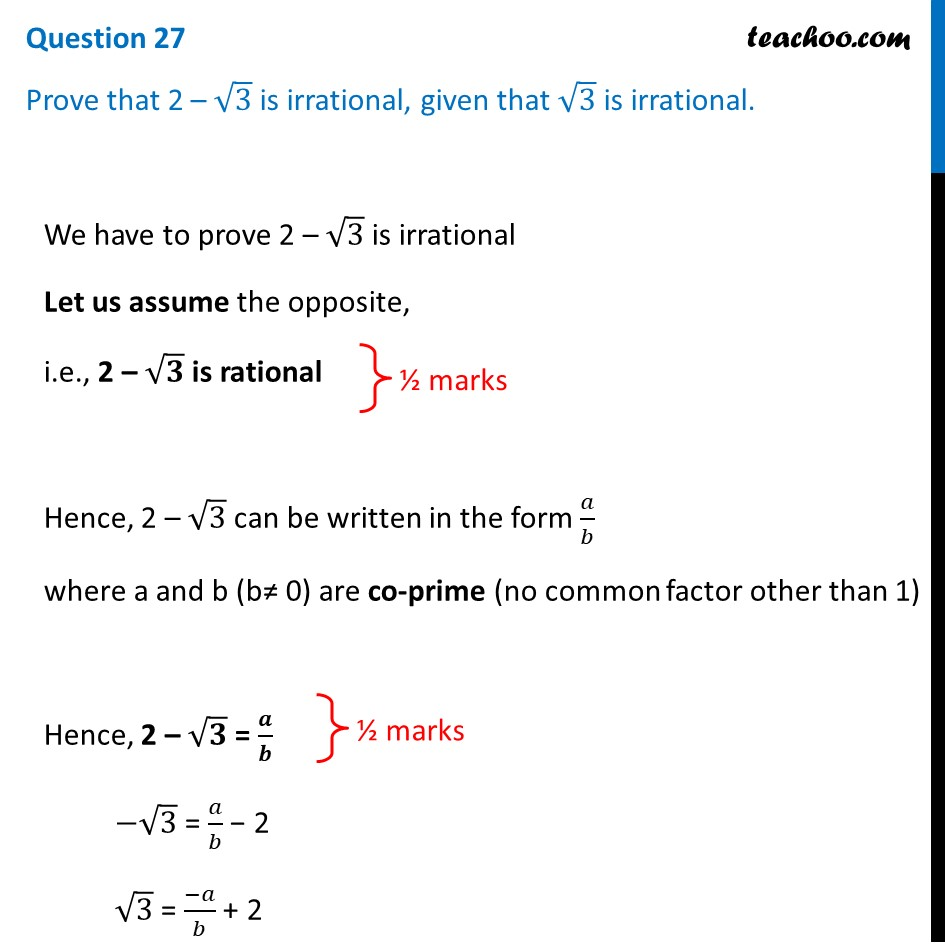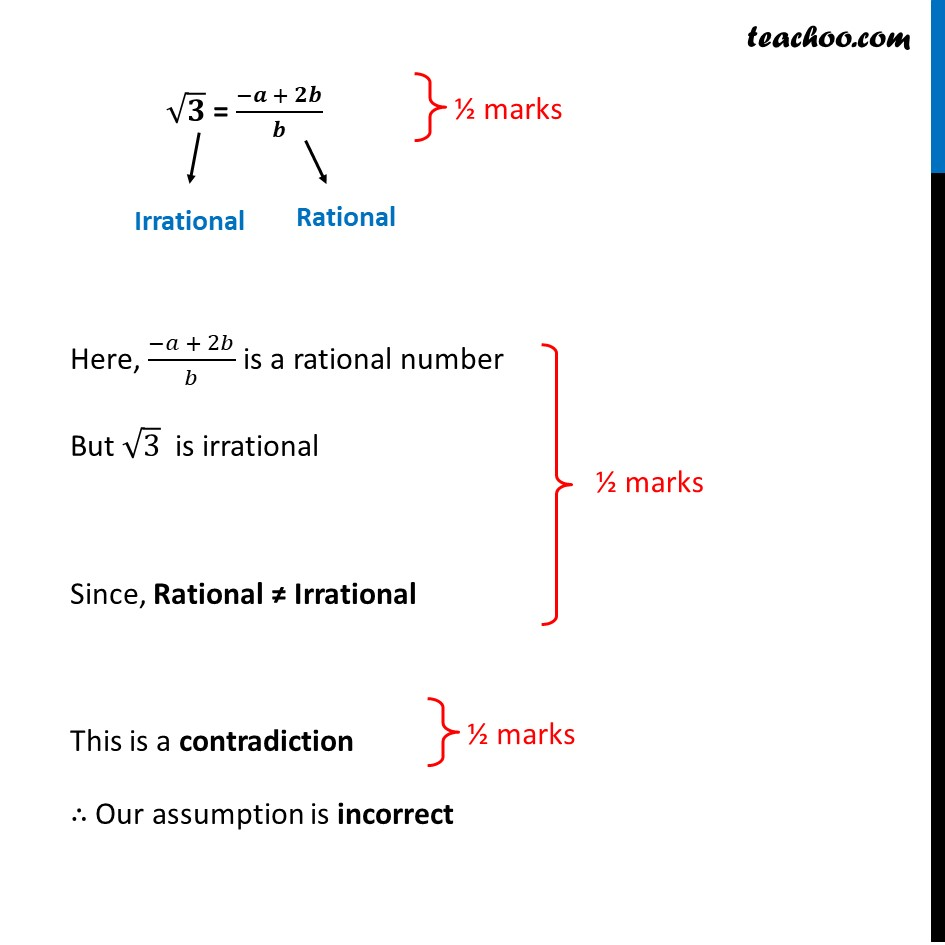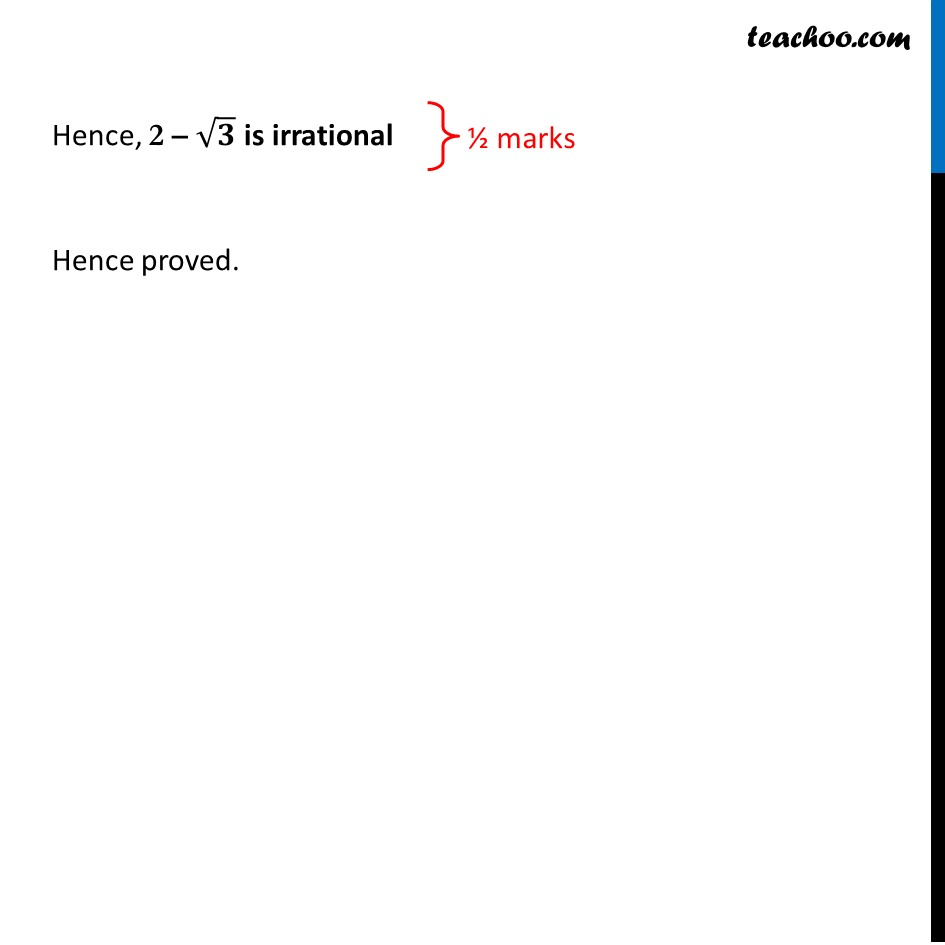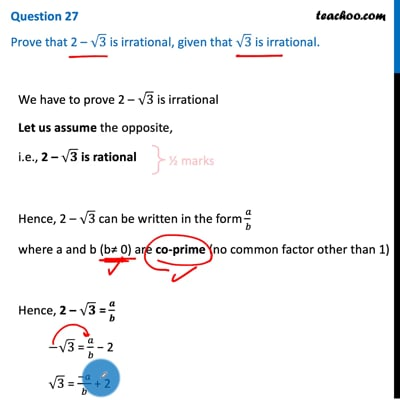CBSE Class 10 Sample Paper for 2021 Boards - Maths Standard

Class 10
Solutions of Sample Papers for Class 10 Boards

## Prove that 2 – √3 is irrational, given that √3 is irrational.This video is only available for Teachoo black users

Introducing your new favourite teacher - Teachoo Black, at only ₹83 per month

### Transcript

Question 27 Prove that 2 – √3 is irrational, given that √3 is irrational. We have to prove 2 – √3 is irrational Let us assume the opposite, i.e., 2 – √𝟑 is rational Hence, 2 – √3 can be written in the form 𝑎/𝑏 where a and b (b≠ 0) are co-prime (no common factor other than 1) Hence, 2 – √𝟑 = 𝒂/𝒃 −√3 = 𝑎/𝑏 − 2 √3 = (−𝑎)/𝑏 + 2 Here, (−𝑎 + 2𝑏)/𝑏 is a rational number But √3 is irrational Since, Rational ≠ Irrational This is a contradiction ∴ Our assumption is incorrect Hence, 2 – √𝟑 is irrational Hence proved.